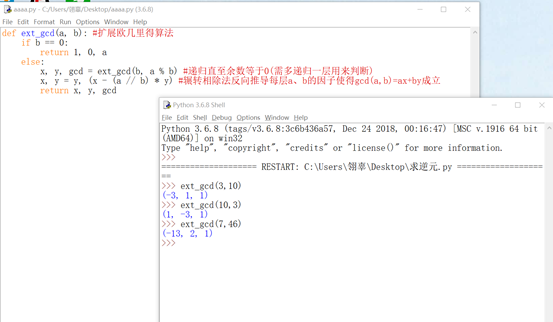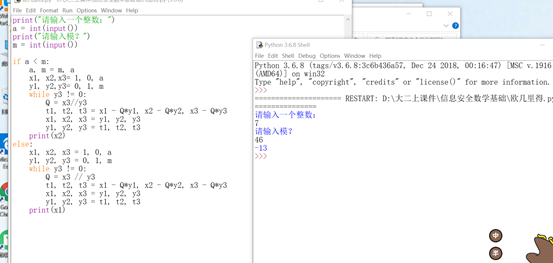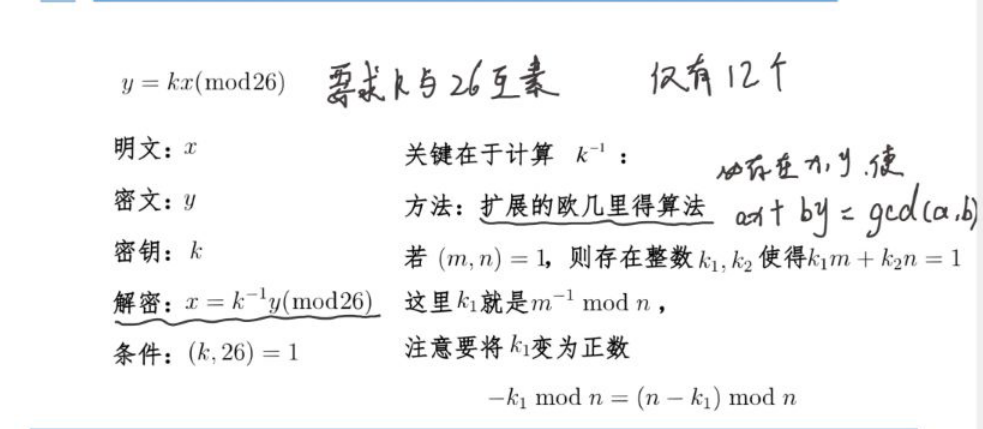• 2021-05-25 03:13:05

关于扩展欧几里得算法和逆元

1.扩欧

a*x1+b*y1=gcd(a,b);

b*x2+(a%b)*y2=gcd(b, (a%b))= gcd(a,b);

a%b=a-(a/b)*b;

联立可得

x1=y2

y1=x2-(a/b)*y2;

递归的边界为b=0

此时x=1,y=0,然后回溯即可。

为什么要x=1,y=0呢？

因为此时gcd(a,b)=gcd(a,0)=a,故a*1+b*0=gcd(a,b)=a;其实y！=0也可以，但是会爆int。

//17.11.6

扩欧的条件是a*x1+b*y1=gcd(a,b)，=右边一定是gcd(a,b),如果gcd(a,b)前面有系数k，在求出来一组解之后，再*k就好了。

//2019.3.26

a*x1+b*y1=c,如果c不是gcd(a,b)的倍数，就没有整数解

怎么求最小正整数解呢

在用扩欧求出一组 ax+by=c 特解之后，比如(x2,y2),对于任意一个通解(x1,y1), ax1+by1=ax2+by2, 移项之后,a(x1-x2)=b(y1-y2),令g=gcd(a,b),a'=a/g,b'=b/g,则a'(x1-x2)=b'(y1-y2), 因为 gcd(a',b')=1,  (x1-x2)=kb' ,同理(y1-y2)=k1 a' ,所以x1=x2+kb',所以最小正整数解就是特解x0的 (x0%b'+b')%b', 这样就不用管特解的正负了。

如果对于读入的a,b,c存在小于0的，令flag=-1,x*=flag就完事了

更多相关内容
• 此为扩展欧几里得算法求乘法逆元的完整程序，图形界面，使用 vc6.0 完成，完全标准正式的格式，绝对值10积分，有完整的代码，请使用 vc6.0 打开 DSW 工程文件，然后就可完全执行。
• 扩展欧几里得算法是欧几里得算法（又叫辗转相除法）的扩展。通常谈到最大公因子时, 我们都会提到一个非常基本的事实: 给予二整数 a 与 b, 必存在有整数 x 与 y 使得ax + by = gcd(a,b)。因此，有两个数a,b，对它们...

扩展欧几里得算法是欧几里得算法（又叫辗转相除法）的扩展。通常谈到最大公因子时, 我们都会提到一个非常基本的事实: 给予二整数 a 与 b, 必存在有整数 x 与 y 使得ax + by = gcd(a,b)。因此，有两个数a,b，对它们进行辗转相除法，可得它们的最大公约数；然后，收集辗转相除法中产生的式子，倒回去，可以得到ax+by=gcd(a,b)的一组整数特解。

以下是扩展欧几里得算法的python实现：

1.递归

#扩展欧几里得算法
def ext_gcd(a, b):
if b == 0:
return 1, 0, a
else:
x, y, gcd = ext_gcd(b, a % b) #递归直至余数等于0(需多递归一层用来判断)
x, y = y, (x - (a // b) * y) #辗转相除法反向推导每层a、b的因子使得gcd(a,b)=ax+by成立
return x, y, gcd



2.非递归

print("请输入一个整数：")
a = int(input())
print("请输入模？")
m = int(input())

if a < m:
a, m = m, a
x1, x2,x3= 1, 0, a
y1, y2,y3= 0, 1, m
while y3 != 0:
Q = x3//y3
t1, t2, t3 = x1 - Q*y1, x2 - Q*y2, x3 - Q*y3
x1, x2, x3 = y1, y2, y3
y1, y2, y3 = t1, t2, t3
print(x2)
else:
x1, x2, x3 = 1, 0, a
y1, y2, y3 = 0, 1, m
while y3 != 0:
Q = x3 // y3
t1, t2, t3 = x1 - Q*y1, x2 - Q*y2, x3 - Q*y3
x1, x2, x3 = y1, y2, y3
y1, y2, y3 = t1, t2, t3
print(x1)



以下是两种方法的运行验证结果说明以上代码正确有效。

展开全文python 信息安全
• 根据扩展欧几里得算法实现了对输入的一个数字取乘法逆元
• 欧几里得算法：又叫做辗转相除法，用来两个数的最大公约数。通过辗转相除，当余数为0的时候，最后的除数就是两个数的最大公约数。 例如：20和11的最大公约数 每次将除数作为下一个式子的被除数，将余数作为...

## 欧几里得算法

背景知识：

欧几里得算法：又叫做辗转相除法，用来求两个数的最大公约数。通过辗转相除，当余数为0的时候，最后的除数就是两个数的最大公约数。

例如：求20和11的最大公约数

每次将除数作为下一个式子的被除数，将余数作为下一个式子的除数

20➗11=1......9

11➗9=1......2

9➗2=4......1

2➗1=2......0

所以最大公约数为最后一个式子的除数1，即gcd(20，11)=1

## 扩展欧几里得算法与逆元

扩展欧几里得算法结论：对整数 a 与 b来说, 必存在整数 x 与 y 使得

ax + by = gcd(a,b)

注：具体证明此处不做展开

逆元定义：ax = 1(mod p)，此时a与x互为逆元

例如a = 4，p = 11，由于4*3=12,12 mod 11 = 1,a=4在模11条件下的逆元为x=3。

为什么扩展欧几里得算法可以求解逆元：

由逆元定义可知满足ax%p=1，因此a与b互为逆元时，加上k倍的p也不影响，毕竟kp对p取余之后为0,所以可以将求逆元的过程转换成：ax+kp=1,此时a与p已知，这就成功转化成扩展欧几里得算法求解的形式，我们可以求出x和k，x即为a对应的逆元。

对于复杂式子我们不能直接看出对应的逆元，具体操作步骤如下所示。

例如：求11在(mod20)条件下的逆元

上个例子中求得：gcd(20，11)=1

代入扩展欧几里得式子：

11x+20y=1

具体求解方法如下所示（类似于欧几里得算法求解过程）：

20=11*1+9                               ----------①

11=9*1+2                                 ----------②

9=2*4+1                                  ----------③

写成上述的形式是类似于计算机求解过程，因此下面的移向操作并不是多此一举。

由式子①，②（移项）可得：

20-11*1=9                                  ----------④

11-9*1=2                                    ----------⑤

④带入⑤（目的是消去9，当求解过程中有很多式子时，就是这样一步步消去中间项）

11-（20-11*1）*1=2                   ----------⑥

再将④和⑥代入③消去中间值9和2，可得：

20-11*1=（11-（20-11*1）*1）*4+1

合并同类项可得：

5*20-9*11=1

也就解除了最开始的式子11x+20y=1，得到x=-9,y=5

总结：对式子ax = 1(mod p)我们已知a和p，先求p=a*x1+p1（此时的p1实际上时p与a运算的余数）,然后将p=a,a=p1代入式子不断进行迭代，直到计算出余数为1时，pi=a*xi+1,就可以不断移向消去中间项，最后计算出对应的逆元。

## 乘法密码答疑：

图中是以英文字母为例，假设26个字母对应数字0-25

1.为什么k要与26互素

因为互素的条件下才能将0-25与k运算之后均匀的映射到每一个位置，否则肯定会有重叠的部分。

2.怎么求k的逆元

由于k与26互素，所以gcd（k，26）=1.

已知k和p=26，利用扩展欧几里得算法：kx+py=1可求得x,y，此时x即为对应的逆元。

展开全文• 师父的扩展欧几里得算法详细博客师父哟大神的求逆元详细博客大神的呢gcd(a,b)即a和b的最大公约。用辗转相除法求得。扩展欧几里得算法是欧几里得算法(又叫辗转相除法)的扩展。除了计算a、b两个整数的最大公约数，此...

师父的扩展欧几里得算法详细博客师父哟

大神的求逆元详细博客大神的呢

gcd(a,b)即求a和b的最大公约。用辗转相除法求得。

扩展欧几里得算法是欧几里得算法(又叫辗转相除法)的扩展。除了计算a、b两个整数的最大公约数，此算法还能找到整数x、y(其中一个很可能是负数)。通常谈到最大公因子时, 我们都会提到一个非常基本的事实: 给予二整数 a 与 b, 必存在有整数 x 与 y 使得ax + by = gcd(a,b)。有两个数a,b，对它们进行辗转相除法，可得它们的最大公约数–这是众所周知的。然后，收集辗转相除法中产生的式子，倒回去，可以得到ax+by=gcd(a,b)的整数解。

ex_gcd(a,b,x,y)

假设a>b;

1、若b=0,则gcd(a,b)=a,得到x=1,y=0;

2、若ab!=0

有ax1+by1=gcd(a,b);

bx2+(a%b)y2=gcd(b,a%b);

由欧几里得算法可得：gcd(a,b)=gcd(b,a%b);

即有：

ax1+by1=bx2+(a%b)y2;

即，ax1+by1=bx2+[a-(a/b)b]y2=ay2+bx2-b(a/b)y2;

由于 a和b是定值，且等式恒等，所以，

x1=y2,

y1=x2-(a/b)y2;

这样通过求解x2,y2来得到x1,y1。

代码如下：

int ex_gcd(int a,int b,int &x,int &y) //扩展欧几里得

{

if(b==0)

{

x=1;

y=0;

return a;

}

int r=ex_gcd(b,a%b,x,y);

int t=x;

x=y;

y=t-a/b*y;

return r;

}

此算法即可求出gcd(a,b)，也可求出x和y。

乘法逆元：对于缩系中的元素，每个数a均有唯一的与之对应的乘法逆元x,使得ax≡1(mod n)。一个数有逆元的充分必要条件是gcd(a,n)=1,此逆元唯一存在。

逆元的含义：模n意义下，1个数a如果有逆元x，那么除以a相当于乘以x。

扩展欧几里得算法求乘法逆元：

给定模数n，求a的逆相当于求解ax=1(mod n),这个方程可以转化为ax-my=1,然后套用二元一次方程的方法，用扩展欧几里得算法求得一组x0,y0和gcd;检查gcd是否为1

gcd不为1说明逆元不存在，若为1,调整x0到0~m-1的范围中即可。

代码如下：

int mod_reverse(int a,int n)//ax=1(mod n) 求a的逆元x

{

int d,x,y;

d=ex_gcd(a,n,x,y);

if(d==1)

return (x%n+n)%n;

else

return -1;

}

还需要更加加深理解。

展开全文• 案例：gcd(45,257) step1:gcd(45,257)=gcd(45,45*5+32)=gcd(32,45)=...=gcd(13,13*3+6)=gcd(6,13)=gcd(6,2*6+1)=gcd(1,6)=1，所以45关于257存在乘法逆元 step2:1=13-（6*2）=13-（（32-2*13）*2）=13*5-32*2=（4...
• 这样就可以用扩展欧几里得算法求x了。 注意：在gcd不为1说明逆元不存在（因为c=1，c%gcd==0为有整数解的充分必要条件），若为1,调整x0到0~m-1的范围中即可 int ex_gcd(int a,int b,int &amp;x,int &amp;...
• 用递归实现的求逆元代码，使用中的算法扩展欧几里得算法。输入本元和模数，得到乘法逆元
• a关于模b的乘法逆元 package main import “fmt” func main(){ var s int var n int fmt.Scan(&s) fmt.Scan(&n) var x,y *int x=new(int) y=new(int) *x=0 *y=0 var d int=Exgcd(s,n,x,y) fmt....go语言
• 裴蜀定理 ： 有一个线性不定方程ax+by=c 若此方程有解，那么 c=k*gcd(a,b) ，k 为任意正整数 证明： 以下 gcd(a,b) 简称 gcd (ax+by) mod gcd=0 ...扩展欧几里得算法： 如何求解上述方程： 因为涉及...
• 扩展欧几里得算法求逆元）总结 1、在RSA算法生成私钥的过程中涉及到了扩展欧几里得算法（简称exgcd），用来求解模的逆元。 2、首先引入逆元的概念： 逆元是模运算中的一个概念，我们通常说 A 是 B 模 C 的...
• Python实现扩展欧几里得算法求乘法逆元 1. 扩展欧几里得算法 已知整数a、b，扩展欧几里得算法可以在求得a、b的最大公约数的同时，能找到整数x、y（其中一个很可能是负数），使它们满足贝祖等式 ax+by=gcd(a,b) ax+by...
• 1、在RSA算法生成私钥的过程中涉及到了扩展欧几里得算法（简称exgcd），用来求解模的逆元。 2、首先引入逆元的概念： 逆元是模运算中的一个概念，我们通常说 A 是 B 模 C 的逆元，实际上是指 A * B = 1 mod C，...
• 来了来了，最难搞的算法终于还是来了，在了解 Web 通信的时候我一度感觉自己变成了通信专业的，现在又感觉自己是一个数统院的学生； 欧几里得算法： ...定义：扩展欧几里得算法实现了对以下方程求解
• 扩展欧几里得算法： ax+by=gcd(a,b)​ax+by=gcd(a,b)​ax+by=gcd(a,b)​ 出满足条件唯一的x,y的值 设：设：设： r0=q1∗r1+r2r_{0}=q_{1}*r_{1}+r_{2}r0​=q1​∗r1​+r2​ r1=q2∗r2+r3r_{1}=q_{2}*r_{2}+r_{3}r1...
• 扩展欧几里得算法乘法逆元 扩展的欧几里德算法可用于求解a mod b的逆元，而逆元求解在RSA加密算法中是不可缺少的一步 伪代码如下： def fangshe_reverse(a,b): X=[0,1] Y=[1,0] X.append(int(a)) Y.append...python 古典密码
• 代码：扩展欧几里得求逆元代码 #include #include using namespace std; int ext_gcd(int a,int b,int &x,int &y){//扩展欧几里得求,一组解x,y if(!b) {x=1,y=0;return a;} int d=ext_gcd(b,a%b,y,x)...
• 扩展欧几里得算法求逆元 解方程 ax + by = gcd(a, b) 在欧几里得算法中通过定理gcd(a, b) = gcd(b, a%b)，我们使用递归求得a与b的最大公约数，在递归边界当b = 0时，a = gcd(a, b)，此时显然有a * 1 + b * 0 = gcd。...
• 文章目录一、解析二、思路三、代码1、Main2、Poly_GCD3、Poly_Division4、Poly_Multi5、Poly_Multi_Eye 一、解析 Poly_GCD(r1,r2,nums)： 接收多项式r1,r2(r1>=r2),以及多项式长度nums 返回其每一步的商组成的...
• 欧几里德有个十分又用的定理： gcd(a, b) = gcd(b , a%b) ，这样，我们就可以在几乎是 log 的时间复杂度里求解出来 a 和 b 的最大公约数了，这就是欧几里德算法。例如：gcd(15750, 27216) = gcd(15750, 1
• 给定两个正整数m和n,我们计算它们的最大公因子d和两个整数a和b,使得a*m+b*n=d...算法欧几里得算法完全一样,为计算最大公约数的算法. 　最终要求的为a*m+b*n=d=GCD(m,n);如果改式子成立由欧几里得算法可推出a’*n+b’*
• 欧几里德算法又称辗转相除法，用于计算两个整数a,b的最大公约数。 gcd函数就是用来(a,b)的最大公约数的。 gcd函数的基本性质： gcd(a,b)=gcd(b,a)=gcd(-a,b)=gcd(|a|,|b|) 公式表述 gcd(a,b)=gcd(b,a mod b)...
• 0. 欧几里得算法 欧几里得算法用于求解两个数的最大公约数。代码如下: int gcd(int a, int b){ if(b == 0) return a; return gcd(b, a % b); } 当b为0时，结束递归，此时a即为a和b的最大公约数。 1. 裴蜀定理 ...
• 原理：1.m是正整数，r属于Zm，且gcd(r,m)=1,存在s属于Zm，使得...3.因为由1知，r和m互素，所以gcd(r,m)=1，则可以使用扩展欧几里得算法求得x和y，则等式ax+by=1成立。步骤：1.输入两个数a,b;a>=b;2.若b=0,则d=a,......